Related Articles

# Python | Pandas dataframe.eq()

• Last Updated : 19 Nov, 2018

Python is a great language for doing data analysis, primarily because of the fantastic ecosystem of data-centric python packages. Pandas is one of those packages and makes importing and analyzing data much easier.

Pandas` dataframe.eq()` is a wrapper used for the flexible comparison. It provides a convenient way to perform comparison of dataframe object with constant, series or another dataframe object.

Syntax: DataFrame.eq(other, axis=’columns’, level=None)

Parameters:
other : Series, DataFrame, or constant
axis : {0, 1, ‘index’, ‘columns’}
level : None by default

Returns: result : DataFrame containing boolean values

Example #1: Use `eq()` function to find the result of comparison between a dataframe and a constant.

 `# importing pandas as pd``import` `pandas as pd`` ` `# Creating the dataframe with NaN value``df ``=` `pd.DataFrame({``"A"``:[``5``, ``3``, ``None``, ``4``],``                   ``"B"``:[``None``, ``2``, ``4``, ``3``],``                   ``"C"``:[``4``, ``3``, ``8``, ``5``],``                   ``"D"``:[``5``, ``4``, ``2``, ``None``]})`` ` `# Print the dataframe``df`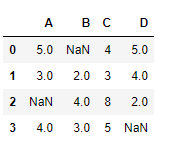Now find the comparison of the dataframe element with value 2.

 `# To find the comparison result``df.eq(``2``)`

Output :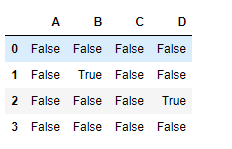The output is a dataframe with cells containing the result of the comparison. True value indicating that the cell value is equal to the comparison value and False indicating non-equal value being compared. Notice, how missing values are evaluated to be false. If we compare two `NaN` using equality operator then the result will be false.

Example #2: Use `eq()` function to test for equality between a data frame object and a series object

 `# importing pandas as pd``import` `pandas as pd`` ` `# Creating the dataframe ``df ``=` `pd.DataFrame({``"A"``:[``5``, ``3``, ``6``, ``4``],``                   ``"B"``:[``11``, ``2``, ``4``, ``3``],``                   ``"C"``:[``4``, ``3``, ``8``, ``5``],``                   ``"D"``:[``5``, ``4``, ``2``, ``8``]})`` ` `# Print the dataframe``df`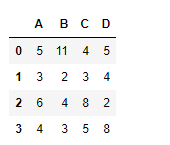Now create a series object with no. of elements equal to the element along the index axis.

Note: If the dimension of the index axis of the dataframe and the series object is not same then an error will occur.

 `# Creating a pandas series object``series_object ``=` `pd.Series([``11``, ``3``, ``4``, ``8``])`` ` `# Print the series_obejct``series_object`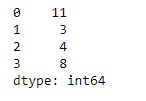Now, find the comparison between the dataframe object and the series object along the index axis. The dimension of the series and the dataframe axis being taken for comparison should be same.

 `# To find the comparison between ``# dataframe and the series object.``df.eq(series_object, axis ``=` `0``)`

Output :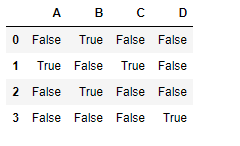The output is a dataframe with cells containing the result of the comparison of the current cell element with the corresponding series object cell.

Attention geek! Strengthen your foundations with the Python Programming Foundation Course and learn the basics.

To begin with, your interview preparations Enhance your Data Structures concepts with the Python DS Course. And to begin with your Machine Learning Journey, join the Machine Learning – Basic Level Course

My Personal Notes arrow_drop_up